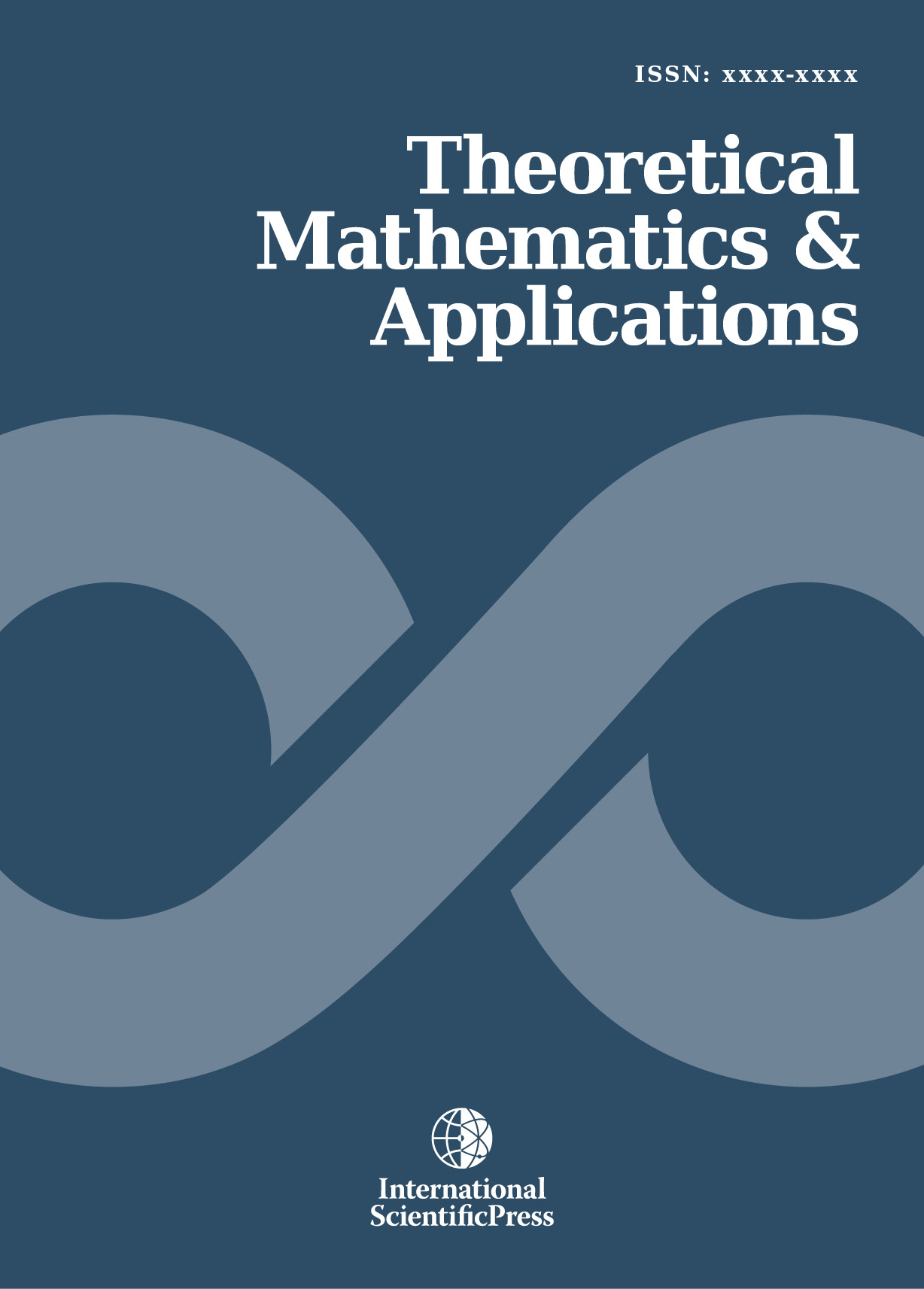# Theoretical Mathematics & Applications

#### Perturbation Least-Squares Chebyshev method for solving fractional order integro-differential equations

•[ Download ]
• Times downloaded: 1043
• Abstract

A numerical scheme based on the perturbation Least-Squares Chebyshev procedure for solving fractional order integro - differential equations is presented in this paper. An approximate solution taken together with the Least - Squares method are utilized to reduce the fractional integro-differential equations to system of algebraic equations, which are solved for the unknown constants associated with the approximate solution. Three numerical examples are considered to demonstrate the accuracy and effectiveness of the method. The results obtained are in good agreement with existing results in literature to a reasonable extent and converge to the exact solutions of the chosen problems when such existed in closed form .ISSN: 1792-9687 (Print)
1792-9709 (Online)